# Parallel Lines Intersected by a Transversal

Anderson Gomes Da Silva, Yuanxin (Amy) Yang Alcocer
• Author
Anderson Gomes Da Silva

Anderson holds a Bachelor's and Master's Degrees (both in Mathematics) from the Fluminense Federal University and the Pontifical Catholic University of Rio de Janeiro, respectively. He was a Teaching Assistant at the University of Delaware (UD) for two and a half years, leading discussion and laboratory sessions of Calculus I, II and III. In the Winter of 2021 he was the sole instructor for one of the Calculus I sections at UD.

• Instructor
Yuanxin (Amy) Yang Alcocer

Amy has a master's degree in secondary education and has been teaching math for over 9 years. Amy has worked with students at all levels from those with special needs to those that are gifted.

Read about transversal lines and the types of transversal angles. Learn about the congruency of angles formed by parallel lines and transversals with examples. Updated: 09/30/2021

Show

## Transversal Lines

A transversal line is a line that intercepts one or more lines. In the image we show {eq}r, s, {/eq} and {eq}t, {/eq} which are pairwise transversal lines, based on the drawing.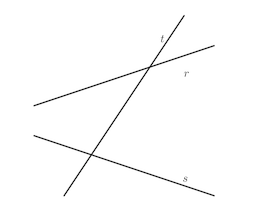Even though the intersection of lines {eq}r {/eq} and {eq}s {/eq} is not shown in the figure, it looks like those two lines are not parallel, and cut each other to the left of {eq}t. {/eq}

### Parallel Lines and Transversals

Parallel lines are two or more coplanar (distinct) lines whose intersection is the empty set. In other words, they do not share a common point. In the next image, we have two lines intercepted by a single transversal.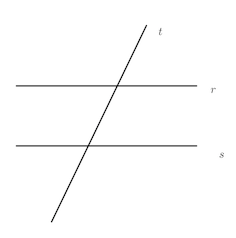However, there is no reason for us to restrict ourselves from having more than two parallel lines or more than one transversal. The following image illustrates this.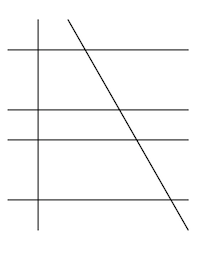As we are going to see, parallel lines and transversals are relevant for the angles they form and by the properties that such angles have. Some angles formed by parallel lines and transversal are congruent and others are supplementary, for example.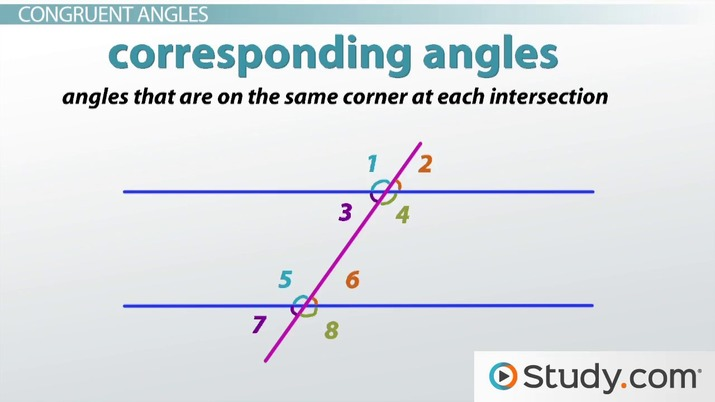An error occurred trying to load this video.

Try refreshing the page, or contact customer support.

Coming up next: Parallel Lines: How to Prove Lines Are Parallel

### You're on a roll. Keep up the good work!

Replay
Your next lesson will play in 10 seconds
• 0:05 Parallel Lines
• 0:37 The Transversal
• 1:03 The Angles
• 1:40 Congruent Angles
• 6:22 Lesson Summary
Save Save

Want to watch this again later?

Timeline
Autoplay
Autoplay
Speed Speed

## What is a Transversal Angle?

Given a pair of transversal lines, the four angles formed by them are called transversal angles. Their properties are going to be the object of study of this lesson when the situation involves parallel lines and transversals and, for this reason, it was important to answer the question "what is a transversal angle?"

### Transversal Angles

When a transversal line cuts a pair of lines, eight angles are formed, out of which some are congruent and some are supplementary. We categorize the transversal angles as corresponding, alternate, vertical and alternate angles. Consider the following image with two parallel lines intercepted by a transversal and the 8 transversal angles that they form.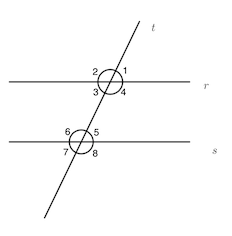### Types of Transversal Angles

Using the last image from the previous section we are going to categorize the types of transversal angles in a list. Two angles can be:

• Corresponding: 1 and 5, 2 and 6, 3 and 7, 4 and 8
• Interior alternate: 3 and 5, 4 and 6
• Exterior alternate: 1 and 7, 2 and 8
• Interior collateral: 4 and 5, 3 and 6
• Exterior collateral: 1 and 8, 2 and 7
• Vertical: 1 and 3, 2 and 4, 5 and 7, 6 and 8

If we move line {eq}r {/eq} until it coincides with {eq}s, {/eq} four angles would be on the top of the other four. This is the idea of the corresponding angles. Alternate angles are the ones on different sides of the transversal, collateral, on the same side, and vertical are the ones that share the vertex, but the rays of one are opposed by the vertex to the rays of the other.

### Congruent Angles Formed by Parallel Lines and Transversals

Some of the angles formed by parallel lines and transversals are congruent, namely: corresponding, interior alternate, exterior alternate, and vertical angles. Let's see why this happens. Because of how we described corresponding angles, it is clear that they are congruent. If we move one of the parallel lines until it coincides with the other, four angles would overlap the other four, and the pairs of overlapping angles are congruent. Now, to see why vertical lines are congruent, consider the two lines depicted in the sequence.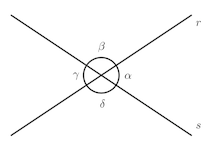To unlock this lesson you must be a Study.com Member.

#### Which straight line is called the transversal line?

A line is said to be a transversal to another one if they intersect at one point. This property is symmetric, meaning that if line r is transversal to line s, then s is transversal to r.

#### How do you find a transversal angle?

Given two intersecting lines, to find a transversal angle one needs the measure of one of the four angles. Then, The desired angle will be either congruent to its vertical pair or supplementary to a consecutive angle.

#### What are the angles formed by a transversal?

When two lines intersect each other, four angles are formed. Such angles are said to be transversal angles and can be grouped in two sets of congruent angles.

### Register to view this lesson

Are you a student or a teacher?

Back

### Resources created by teachers for teachers

Over 30,000 video lessons & teaching resources‐all in one place.Video lessonsQuizzes & WorksheetsClassroom IntegrationLesson Plans

I would definitely recommend Study.com to my colleagues. It’s like a teacher waved a magic wand and did the work for me. I feel like it’s a lifeline.

Jennifer B.
TeacherCreate an account to start this course today
Used by over 30 million students worldwide